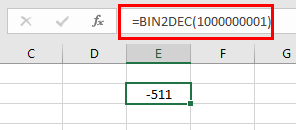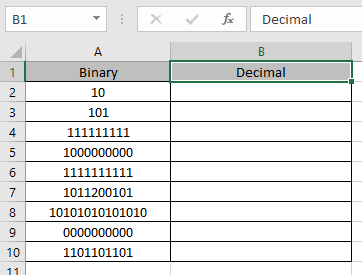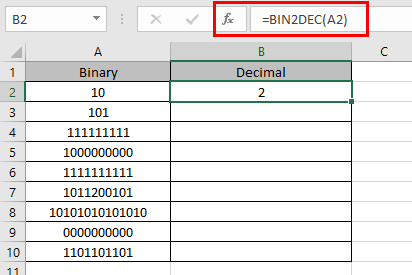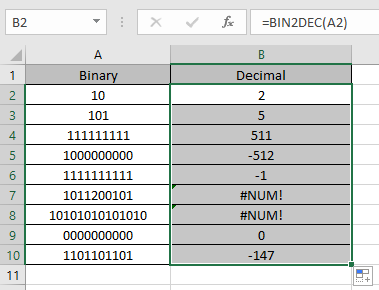# How to use the BIN2DEC Function in Excel

BIN2DEC function in excel is used to convert binary representation of numbers of radix 2 to decimal numbers (radix =10).
The table shown below shows you some of the most used base & their radix of Alpha - numeric characters

 Base radix Alpha-Numeric Characters Binary 2 0 - 1 Octal 8 0 - 7 Decimal 10 0 - 9 hexadecimal 16 0 - 9 & A - F hexatridecimal 36 0 - 9 & A - Z

Binary number is representation of a number of radix 2. Only 2 digits are used in representation of a binary number 0 & 1. Where as decimal number representation have 10 digits from 0, 1, 2, 3, 4, 5, 6, 7, 8, 9 The below table will help you understand better

 Binary Decimal 0000 0 0001 1 0010 2 0011 3 0100 4 0101 5 0110 6 0111 7 1000 8

Conversion formulation is shown below:
Let’s take an example having 4 bits.

0111 (binary) = 23x 0 + 22x 1 + 21x 1 + 20x 1 = 7 (decimal)

The BIN2DEC function converts the binary number of radix 2 to the decimal number of radix 10 (BIN2DEC).

Syntax:

=BIN2DEC ( number )

Number : binary number. Number cannot contain more than 10 characters (10 bits)
The input value to the function cannot contain more than 10 characters ( 10 bits ). Where first number returns the sign of the number (positive or negative) & Other 9 returns the value after the sign. ( 1 for negative & 0 for positive)To get the negative number, input number must have 10 characters starting with 1 as it represents the negative sign. The negative number is calculated using two's complement notation. Any less than 10 characters, the function considers default 0s in front. Any more than 10 characters, the function returns the #NUM! Error. The function returns the max value of 511 & minimum value upto -512 (only integers).

Now let’s get more understanding of the function via using them in some examples.Here we have some binary values in Binary Column. We need to convert these binary numbers to decimal value.

Use the formula in Decimal column:

=BIN2DEC (A2)

A2 : number provided to the function as cell reference

Values to the BIN2DEC function is provided as cell reference.The BIN2DEC representation of 10 of base 2 (binary) is 2 of base 10 (decimal).
Now copy the formula to other cells using the Ctrl + D shortcut key.As you can see here the BIN2DEC function returns the result of the input values.

Notes:

1. Numbers can be given as argument to the function directly without quotes or cell reference in excel.
2. The number must be a valid binary numeric number.
3. If the input number has less than 10 digits, the function takes 0s to the left of the number to complete its 10 character binary value.
4. The function returns the max value of 511 & minimum value upto -512 (only integers).
1. The function returns the #NUM! Error
1. If the input characters exceed by 10 digits.
2. If the input number is not a valid binary expression.
3. If the input value is text or non-numeric.
4. If the input number have fraction part or decimal part for example: 010111.101

Hope you understood how to use BIN2DEC function and referring cell in Excel. Explore more articles on Excel mathematical conversion functions here. Please feel free to state your query or feedback for the above article.

Related Articles:

How to use the DEC2BIN Function in Excel

How to use the BIN2OCT Function in Excel

How to use the BIN2HEX Function in Excel

How to use the DEC2OCT Function in Excel

How to use the DEC2HEX Function in Excel

How to use the OCT2BIN Function in Excel

How to use the OCT2DEC Function in Excel

How to use the OCT2HEX Function in Excel

How to use the HEX2BIN Function in Excel

How to use the HEX2OCT Function in Excel

How to use the HEX2DEC Function in Excel

Popular Articles:

If with wildcards

Vlookup by date

Join first and last name in excel

Count cells which match either A or B

Terms and Conditions of use

The applications/code on this site are distributed as is and without warranties or liability. In no event shall the owner of the copyrights, or the authors of the applications/code be liable for any loss of profit, any problems or any damage resulting from the use or evaluation of the applications/code.